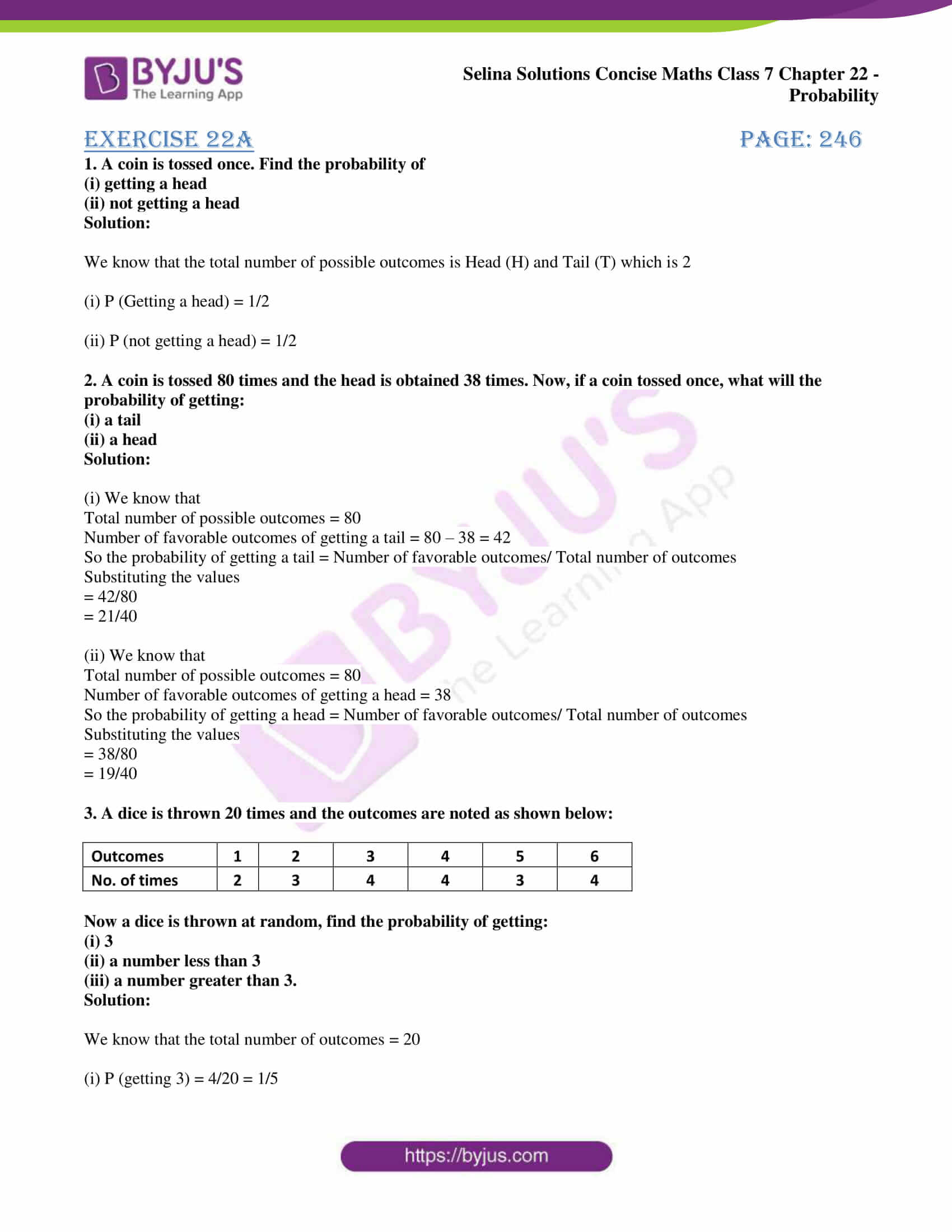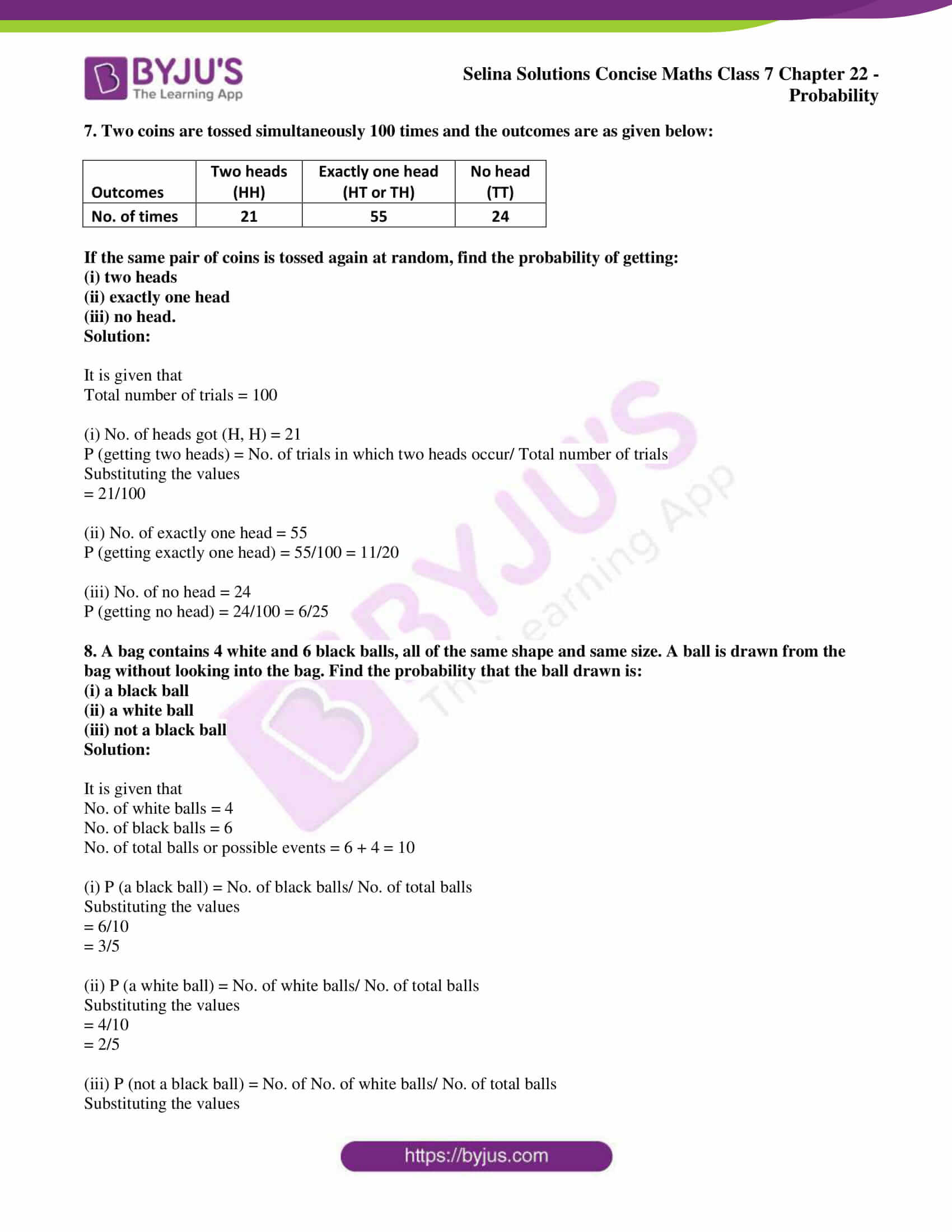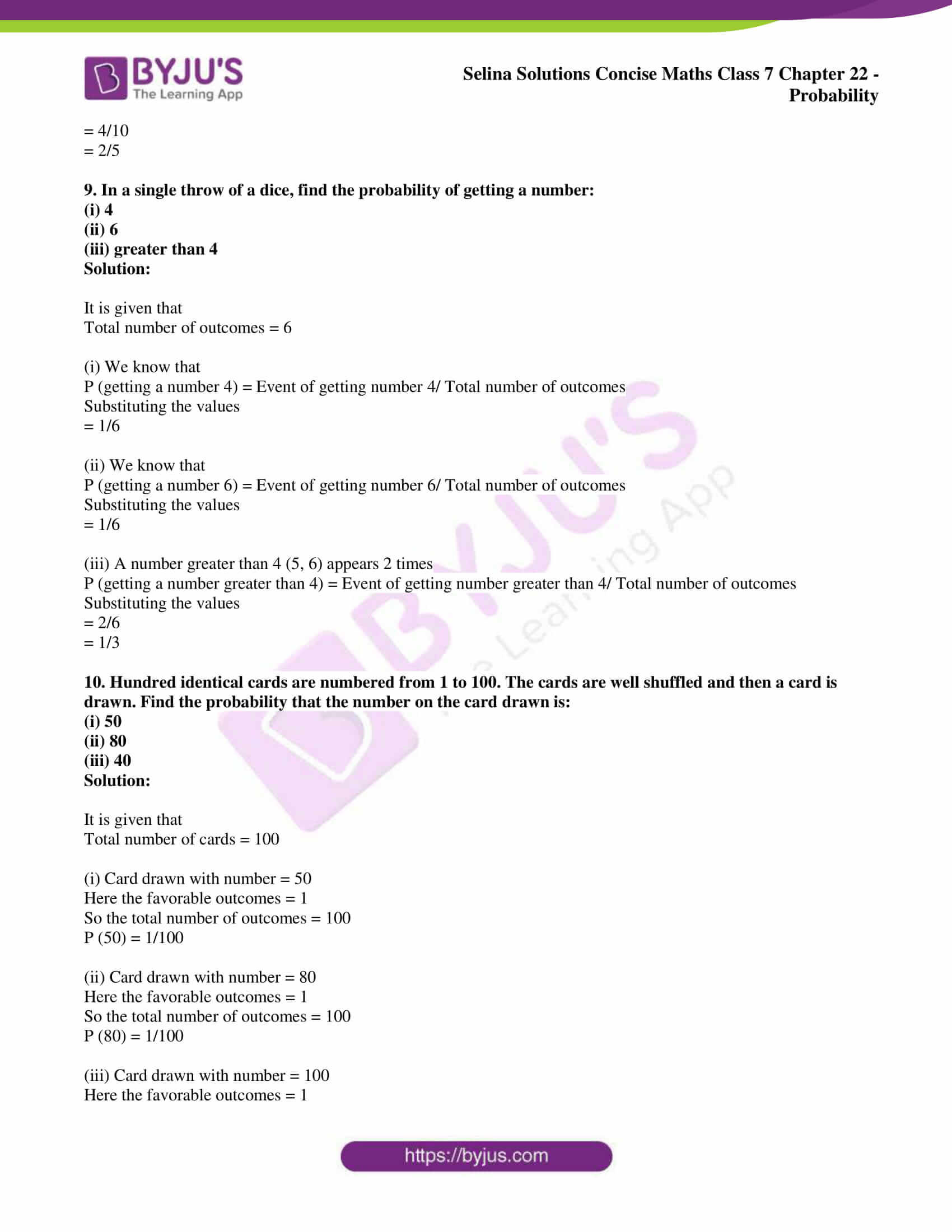# Selina Solutions Concise Maths Class 7 Chapter 22: Probability Exercise 22A

Selina Solutions Concise Maths Class 7 Chapter 22 Probability Exercise 22A help students understand the basic concepts, which are important from the exam perspective. The experimental approach and empirical probability are the major topics which are covered here. The solutions are provided in a step by step format to improve conceptual understanding among the students. For a better performance in the annual exam, students can refer to Selina Solutions Concise Maths Class 7 Chapter 22 Probability Exercise 22A free PDF, from the links which are provided here.

## Selina Solutions Concise Maths Class 7 Chapter 22: Probability Exercise 22A Download PDF### Access Selina Solutions Concise Maths Class 7 Chapter 22: Probability Exercise 22A

#### Exercise 22A page: 246

1. A coin is tossed once. Find the probability of

Solution:

We know that the total number of possible outcomes is Head (H) and Tail (T) which is 2

(i) P (Getting a head) = 1/2

(ii) P (not getting a head) = 1/2

2. A coin is tossed 80 times and the head is obtained 38 times. Now, if a coin tossed once, what will the probability of getting:

(i) a tail

Solution:

(i) We know that

Total number of possible outcomes = 80

Number of favorable outcomes of getting a tail = 80 – 38 = 42

So the probability of getting a tail = Number of favorable outcomes/ Total number of outcomes

Substituting the values

= 42/80

= 21/40

(ii) We know that

Total number of possible outcomes = 80

Number of favorable outcomes of getting a head = 38

So the probability of getting a head = Number of favorable outcomes/ Total number of outcomes

Substituting the values

= 38/80

= 19/40

3. A dice is thrown 20 times and the outcomes are noted as shown below:

 Outcomes 1 2 3 4 5 6 No. of times 2 3 4 4 3 4

Now a dice is thrown at random, find the probability of getting:

(i) 3

(ii) a number less than 3

(iii) a number greater than 3.

Solution:

We know that the total number of outcomes = 20

(i) P (getting 3) = 4/20 = 1/5

(ii) A number less than 3

(1, 2) will appear = 2 + 3 = 5 times

So probability = 5/20 = ¼

(iii) A number greater than 3

(4, 5, 6) will appear = 4 + 3 + 4 = 11 times

So probability = 11/20

4. A survey of 50 boys showed that 21 like tea while 29 dislike it. Out of these boys, one boy is chosen at random. What is the probability that the chosen boy
(i) likes tea
(ii) dislikes tea

Solution:

It is given that

Total number of boys = 50

No. of boys who like tea = 21

No. of boys who dislike tea = 29

(i) Probability of boys who like tea = 21/50

(ii) Probability of boys who dislike tea = 29/50

5. In a cricket match, a batsman hits a boundary 12 times out of 80 balls he plays. Further, if he plays one ball more, what will be the probability that:

(i) he hits a boundary

(ii) he does not hit a boundary

Solution:

It is given that

Total number of balls = 80

No. of times batsman hits a boundary = 12

(i) P (he hits a boundary) = 12/80 = 3/20

(ii) P (he does not hit a boundary) = 68/80 = 17/20

6. There are 8 marbles in a bag with numbers from 1 to 8 marked on each of them. What is the probability of drawing a marble with number

(i) 3

(ii) 7

Solution:

It is given that

Total number of marbles = 8

(i) P (drawing a marble with number 3) = 1/8

(ii) P (drawing a marble with number 7) = 1/8

7. Two coins are tossed simultaneously 100 times and the outcomes are as given below:

 Outcomes Two heads (HH) Exactly one head (HT or TH) No head (TT) No. of times 21 55 24

If the same pair of coins is tossed again at random, find the probability of getting:

Solution:

It is given that

Total number of trials = 100

(i) No. of heads got (H, H) = 21

P (getting two heads) = No. of trials in which two heads occur/ Total number of trials

Substituting the values

= 21/100

(ii) No. of exactly one head = 55

P (getting exactly one head) = 55/100 = 11/20

(iii) No. of no head = 24

P (getting no head) = 24/100 = 6/25

8. A bag contains 4 white and 6 black balls, all of the same shape and same size. A ball is drawn from the bag without looking into the bag. Find the probability that the ball drawn is:

(i) a black ball

(ii) a white ball

(iii) not a black ball

Solution:

It is given that

No. of white balls = 4

No. of black balls = 6

No. of total balls or possible events = 6 + 4 = 10

(i) P (a black ball) = No. of black balls/ No. of total balls

Substituting the values

= 6/10

= 3/5

(ii) P (a white ball) = No. of white balls/ No. of total balls

Substituting the values

= 4/10

= 2/5

(iii) P (not a black ball) = No. of No. of white balls/ No. of total balls

Substituting the values

= 4/10

= 2/5

9. In a single throw of a dice, find the probability of getting a number:

(i) 4

(ii) 6

(iii) greater than 4

Solution:

It is given that

Total number of outcomes = 6

(i) We know that

P (getting a number 4) = Event of getting number 4/ Total number of outcomes

Substituting the values

= 1/6

(ii) We know that

P (getting a number 6) = Event of getting number 6/ Total number of outcomes

Substituting the values

= 1/6

(iii) A number greater than 4 (5, 6) appears 2 times

P (getting a number greater than 4) = Event of getting number greater than 4/ Total number of outcomes

Substituting the values

= 2/6

= 1/3

10. Hundred identical cards are numbered from 1 to 100. The cards are well shuffled and then a card is drawn. Find the probability that the number on the card drawn is:

(i) 50

(ii) 80

(iii) 40

Solution:

It is given that

Total number of cards = 100

(i) Card drawn with number = 50

Here the favorable outcomes = 1

So the total number of outcomes = 100

P (50) = 1/100

(ii) Card drawn with number = 80

Here the favorable outcomes = 1

So the total number of outcomes = 100

P (80) = 1/100

(iii) Card drawn with number = 100

Here the favorable outcomes = 1

So the total number of outcomes = 100

P (100) = 1/100